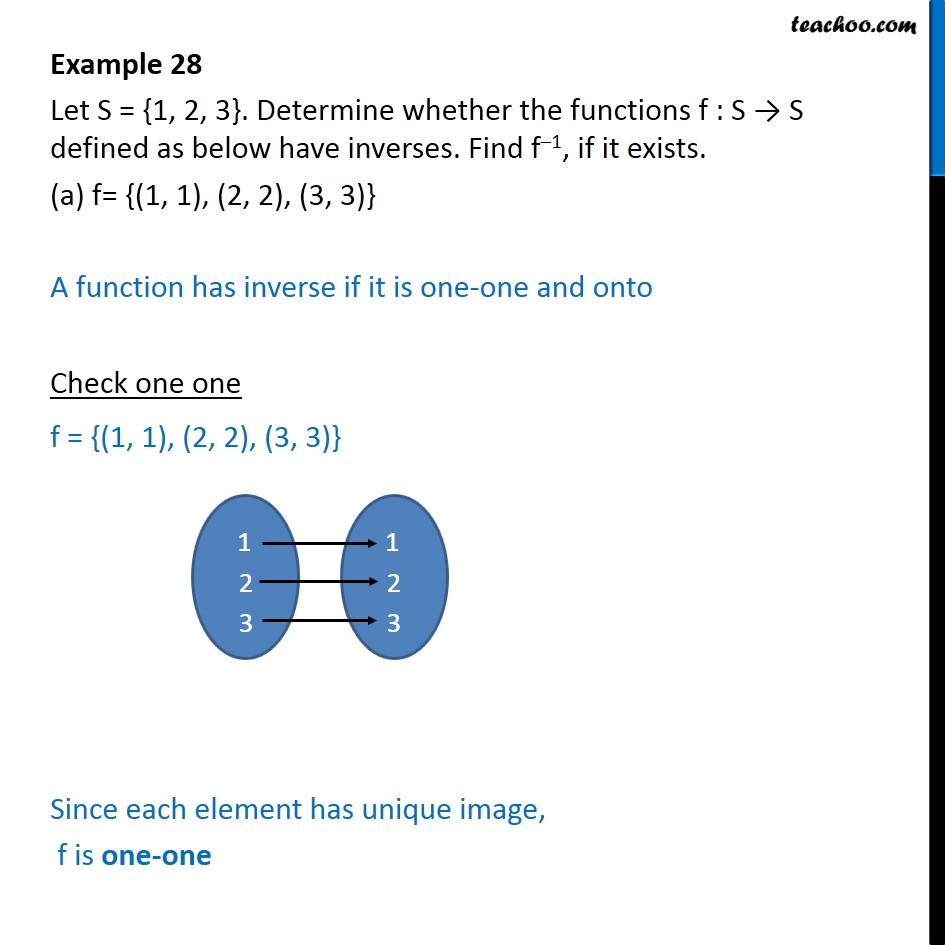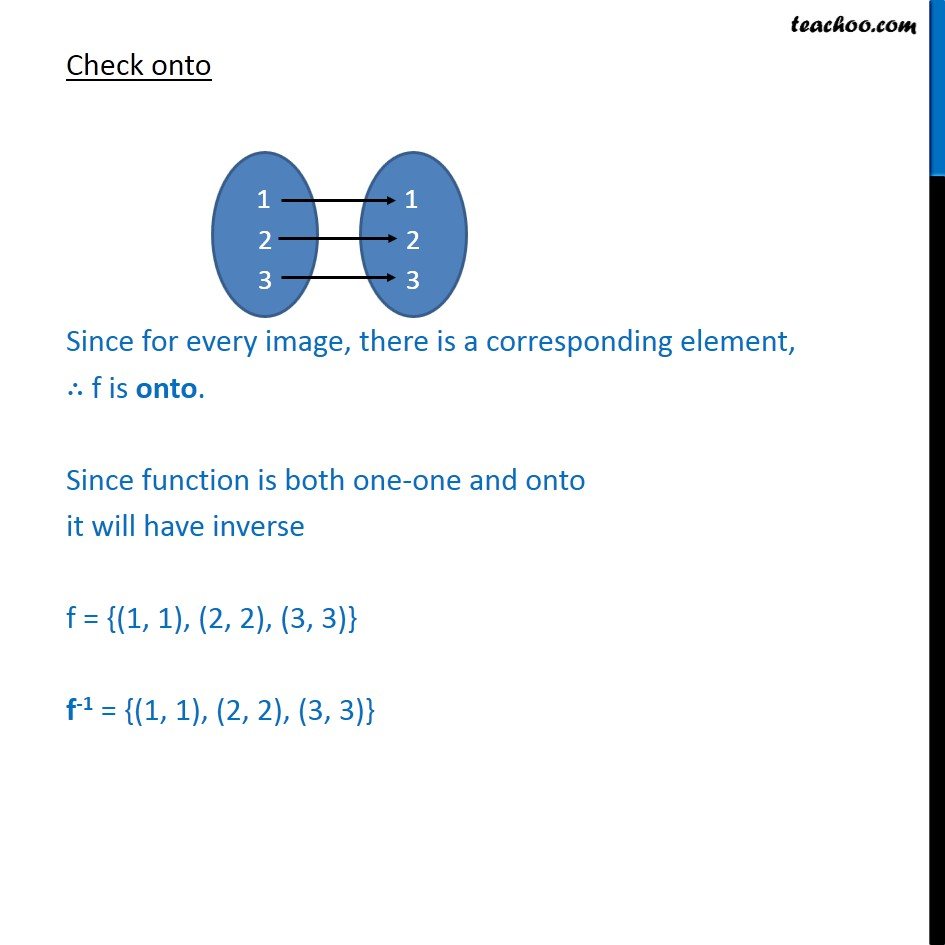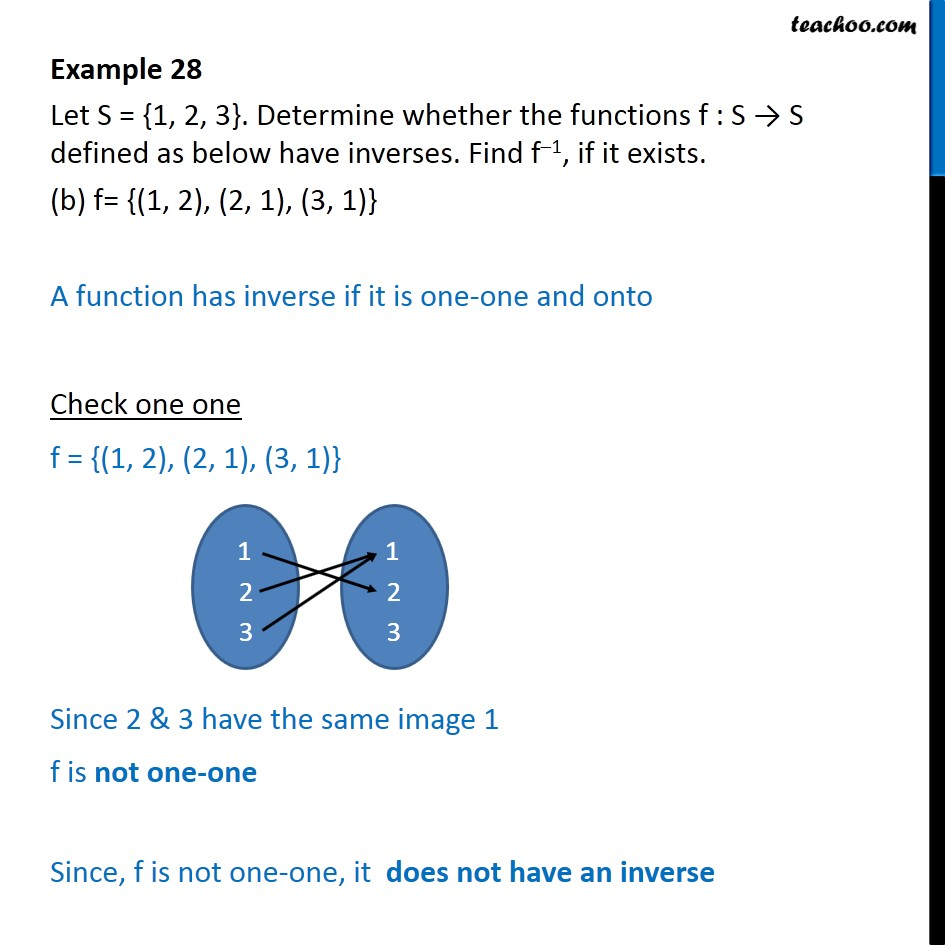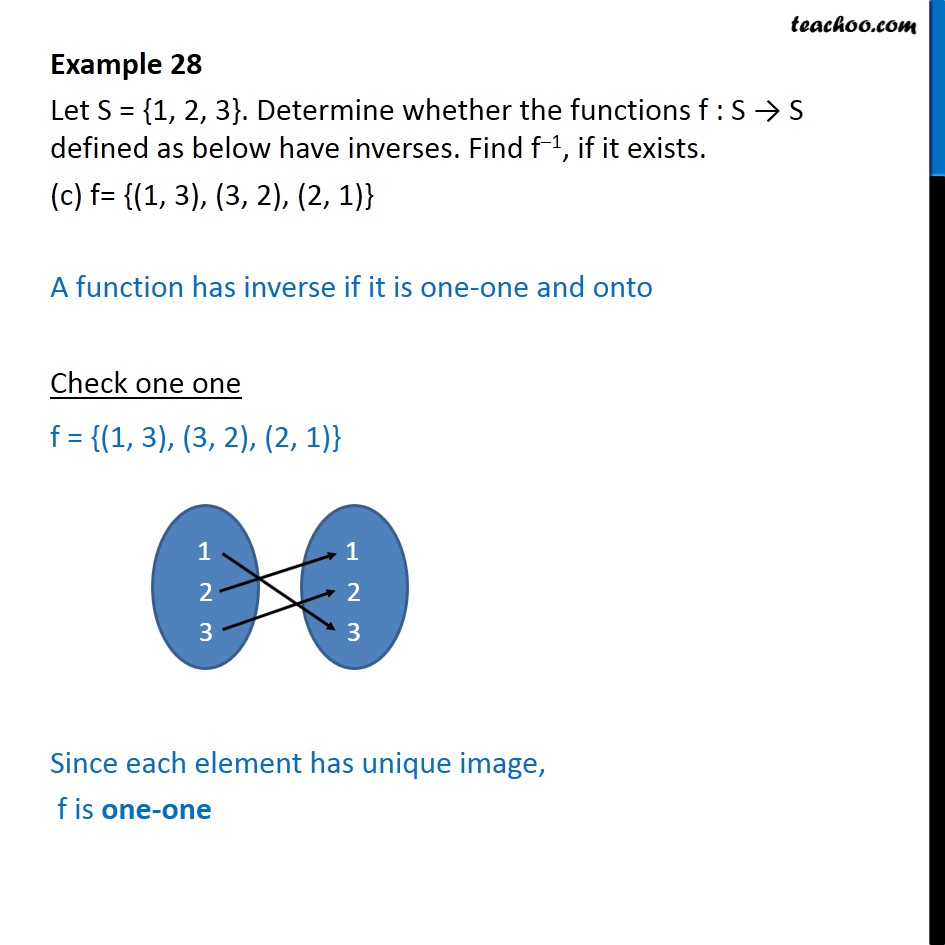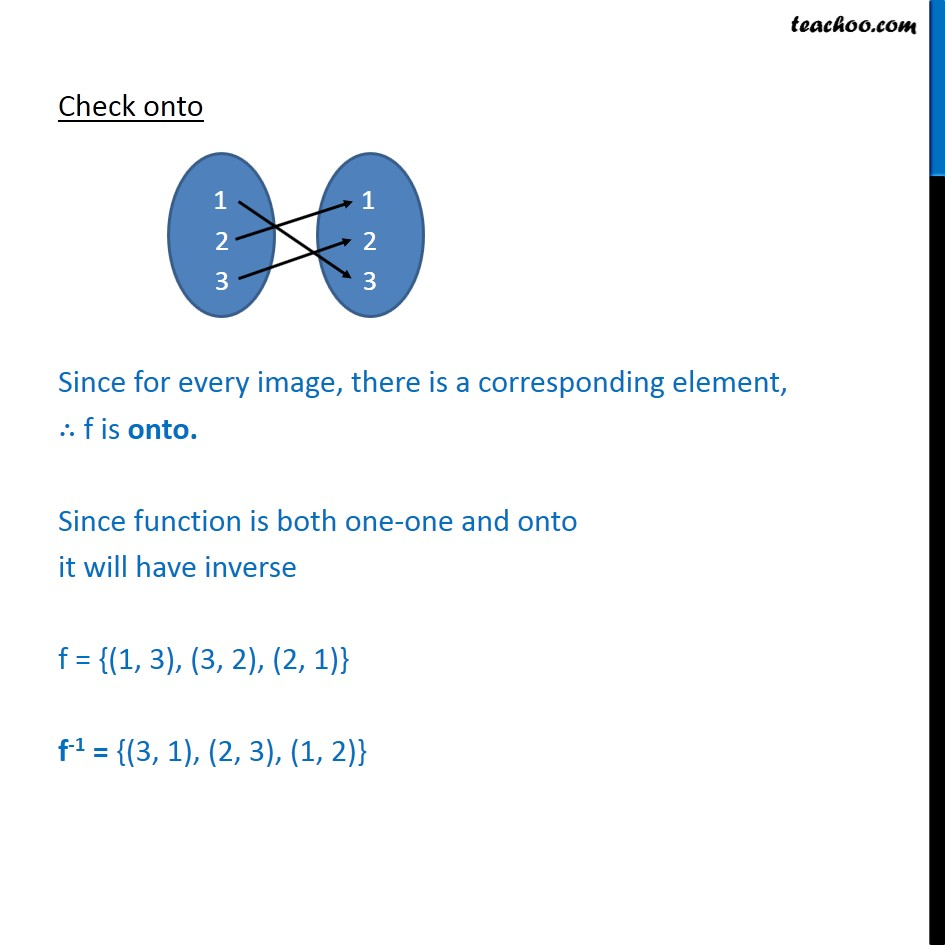Subscribe to our Youtube Channel - https://you.tube/teachoo

1. Chapter 1 Class 12 Relation and Functions
2. Concept wise
3. Finding Inverse

Transcript

Example 28 Let S = {1, 2, 3}. Determine whether the functions f : S → S defined as below have inverses. Find f–1, if it exists. (a) f= {(1, 1), (2, 2), (3, 3)} A function has inverse if it is one-one and onto Check one one f = {(1, 1), (2, 2), (3, 3)} Since each element has unique image, f is one-one Check onto Since for every image, there is a corresponding element, ∴ f is onto. Since function is both one-one and onto it will have inverse f = {(1, 1), (2, 2), (3, 3)} f-1 = {(1, 1), (2, 2), (3, 3)} Example 28 Let S = {1, 2, 3}. Determine whether the functions f : S → S defined as below have inverses. Find f–1, if it exists. (b) f= {(1, 2), (2, 1), (3, 1)} A function has inverse if it is one-one and onto Check one one f = {(1, 2), (2, 1), (3, 1)} Since 2 & 3 have the same image 1 f is not one-one Since, f is not one-one, it does not have an inverse Example 28 Let S = {1, 2, 3}. Determine whether the functions f : S → S defined as below have inverses. Find f–1, if it exists. (c) f= {(1, 3), (3, 2), (2, 1)} A function has inverse if it is one-one and onto Check one one f = {(1, 3), (3, 2), (2, 1)} Since each element has unique image, f is one-one Check onto Since for every image, there is a corresponding element, ∴ f is onto. Since function is both one-one and onto it will have inverse f = {(1, 3), (3, 2), (2, 1)} f-1 = {(3, 1), (2, 3), (1, 2)}

Finding Inverse